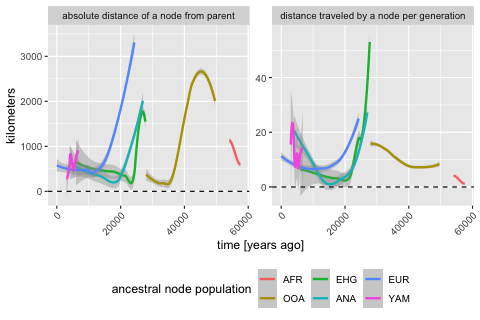# Spatially annotated tree sequences

## Introduction

The main selling point of the slendr R package is programming complex spatially explicit population genetic models. Because we use SLiM as the simulation engine, we can store simulated data efficiently in a tree sequence format which allows us to run large population-scale simulations. In the previous vignettes, we described how you can specify spatial population dynamics and how you can access tree sequence data and calculate population genetic statistics on it (focusing on non-spatial models for simplicity). Now it’s time to show you how to work with simulated tree sequence in a spatial context.

## Model specification

Let’s first load all required R libraries:

library(slendr)

library(dplyr)
library(ggplot2)

seed <- 314159
set.seed(seed)

We begin by specifying our spatial model. We will use the same demographic model of modern human history in West Eurasia, which we extensively discussed in the introductory tutorial and on the main landing page. Here is a complete model definition script, without further comments:

# simulated world map
map <- world(
xrange = c(-15, 60),
yrange = c(20, 65),
crs = "EPSG:3035"
)

# couple of broad geographic regions
africa <- region("Africa", map, polygon = list(c(-18, 20), c(40, 20), c(30, 33),
c(20, 32), c(10, 35), c(-8, 35)))
europe <- region("Europe", map, polygon = list(c(-8, 35), c(-5, 36), c(10, 38),
c(20, 35), c(25, 35), c(33, 45),
c(20, 58), c(-5, 60), c(-15, 50)))
anatolia <- region("Anatolia", map, polygon = list(c(28, 35), c(40, 35), c(42, 40),
c(30, 43), c(27, 40), c(25, 38)))

# define population histories

# African ancestral population
afr <- population(
"AFR", parent = "ancestor", time = 52000, N = 3000,
map = map, polygon = africa
)

# population of the first migrants out of Africa
ooa <- population(
"OOA", parent = afr, time = 51000, N = 500, remove = 25000,
center = c(33, 30), radius = 400e3
) %>%
move(
trajectory = list(c(40, 30), c(50, 30), c(60, 40)),
start = 50000, end = 40000, snapshots = 20
)

# Eastern hunter-gatherers
ehg <- population(
"EHG", parent = ooa, time = 28000, N = 1000, remove = 6000,
polygon = list(
c(26, 55), c(38, 53), c(48, 53), c(60, 53),
c(60, 60), c(48, 63), c(38, 63), c(26, 60))
)

# European population
eur <- population(name = "EUR", parent = ehg, time = 25000, N = 2000, polygon = europe)

# Anatolian farmers
ana <- population(
name = "ANA", time = 28000, N = 3000, parent = ooa, remove = 4000,
center = c(34, 38), radius = 500e3, polygon = anatolia
) %>%
expand_range(
by = 2500e3, start = 10000, end = 7000,
polygon = join(europe, anatolia), snapshots = 20
) # expand the range by 2.500 km

# Yamnaya steppe population
yam <- population(
name = "YAM", time = 7000, N = 500, parent = ehg, remove = 2500,
polygon = list(c(26, 50), c(38, 49), c(48, 50),
c(48, 56), c(38, 59), c(26, 56))
) %>%
move(trajectory = list(c(15, 50)), start = 5000, end = 3000, snapshots = 10)

# geneflow events
gf <- list(
gene_flow(from = ana, to = yam, rate = 0.5, start = 6000, end = 5000, overlap = FALSE),
gene_flow(from = ana, to = eur, rate = 0.5, start = 8000, end = 6000),
gene_flow(from = yam, to = eur, rate = 0.75, start = 4000, end = 3000)
)

# compile the spatial model
model <- compile_model(
populations = list(afr, ooa, ehg, eur, ana, yam),
gene_flow = gf,
generation_time = 30, resolution = 10e3,
competition = 130e3, mating = 100e3, dispersal = 70e3
)

As a sanity check that we defined the demography correctly, you can plot a graph summarizing population divergences and geneflow events by calling plot_model(model):

plot_model(model)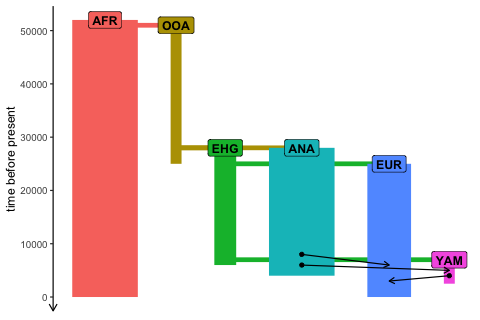And for completeness, here is a (slightly busy) overview of the spatial population ranges that we defined above:

plot_map(afr, ooa, ehg, eur, ana, yam)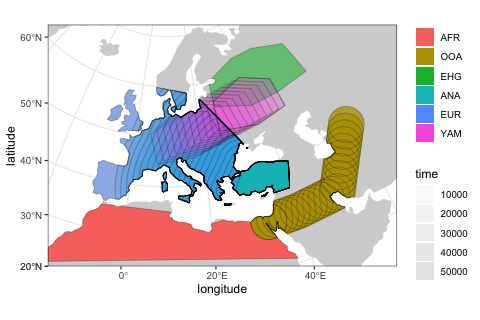## Scheduling sampling events and simulation

Now we will schedule the sampling of a single individual from each population every two thousand years, starting from 40 thousand years ago all the way to the present (this is a feature discussed in the basic tree sequence overview):

# one ancient individual every two thousand years
ancient <- schedule_sampling(model,
times = seq(40000, 1, by = -1000),
list(ooa, 1), list(ehg, 1), list(eur, 1),
list(ana, 1), list(yam, 1))

# present-day Africans and Europeans
present <- schedule_sampling(model, times = 0, list(afr, 5), list(eur, 30))

samples <- rbind(ancient, present)

Finally, we can simulate data from our model and process the output tree sequence (recapitate and simplify it):

ts <- slim(
model, sequence_length = 100e3, recombination_rate = 1e-8, burnin = 200e3,
samples = samples, method = "batch", random_seed = 314159, max_attempts = 1
) %>%
ts_recapitate(recombination_rate = 1e-8, Ne = 10000, random_seed = seed) %>%
ts_simplify()

ts
#> ╔═════════════════════════╗
#> ║TreeSequence             ║
#> ╠═══════════════╤═════════╣
#> ║Trees          │       82║
#> ╟───────────────┼─────────╢
#> ║Sequence Length│   100000║
#> ╟───────────────┼─────────╢
#> ║Time Units     │    ticks║
#> ╟───────────────┼─────────╢
#> ║Sample Nodes   │      250║
#> ╟───────────────┼─────────╢
#> ║Total Size     │144.6 KiB║
#> ╚═══════════════╧═════════╝
#> ╔═══════════╤════╤════════╤════════════╗
#> ╠═══════════╪════╪════════╪════════════╣
#> ║Edges      │ 807│25.2 KiB│          No║
#> ╟───────────┼────┼────────┼────────────╢
#> ║Individuals│ 439│44.7 KiB│         Yes║
#> ╟───────────┼────┼────────┼────────────╢
#> ║Migrations │   0│ 8 Bytes│          No║
#> ╟───────────┼────┼────────┼────────────╢
#> ║Mutations  │   0│ 1.2 KiB│          No║
#> ╟───────────┼────┼────────┼────────────╢
#> ║Nodes      │ 566│21.7 KiB│         Yes║
#> ╟───────────┼────┼────────┼────────────╢
#> ║Populations│   7│ 2.7 KiB│         Yes║
#> ╟───────────┼────┼────────┼────────────╢
#> ║Provenances│   3│36.7 KiB│          No║
#> ╟───────────┼────┼────────┼────────────╢
#> ║Sites      │   0│16 Bytes│          No║
#> ╚═══════════╧════╧════════╧════════════╝

## Extracting spatial tree sequence information

As we showed in the basic tutorial, the most important function for data exploration is ts_nodes(). This function extracts all information about individuals and nodes recorded in a tree sequence object loaded and annotated by slendr :

data <- ts_nodes(ts)

For completeness, we have also functions such as ts_individuals(), ts_nodes() and ts_edges() which extract tree sequence tables in their “raw” unprocessed form, but ts_nodes() is much more convenient for data exploration and analyses. First, it combined information in the low-level tables of individuals and nodes into a single table but more importantly, if the model which generated this data was a spatial model, ts_nodes() automatically annotates the node/individual tables with the position of each node in space (in real projected coordinates) and time. This means that we can do spatial data analysis directly on the table returned by ts_nodes().

Even better, although we can see below that the returned object belongs to slendr’s own class slendr_ts_nodes, it is internally stored as a spatial sf object. This means that we can use all the functionality of the powerful R package sf as well as many other packages for geospatial analyses directly on the data:

class(data)
#>  "slendr"       "slendr_nodes" "sf"           "tbl_df"       "tbl"
#>  "data.frame"

Typing the object into the R console presents a user-friendly summary of the spatio-temporal data extracted from the tree sequence:

data
#> slendr 'nodes' object
#> ---------------------
#> times are expressed in a backward time direction
#>
#> summary of the table data contents:
#>   AFR - 5 'sampled', 5 'remembered', 5 'retained', 5 'alive' individuals
#>   EUR - 54 'sampled', 54 'remembered', 30 'retained', 30 'alive' individuals
#>   OOA - 16 'sampled', 16 'remembered', NA 'retained', 0 'alive' individuals
#>   EHG - 22 'sampled', 22 'remembered', NA 'retained', 0 'alive' individuals
#>   ANA - 24 'sampled', 24 'remembered', NA 'retained', 0 'alive' individuals
#>   YAM - 4 'sampled', 4 'remembered', NA 'retained', 0 'alive' individuals
#>
#> total:
#>   - 125 'sampled' individuals
#>   - 125 'remembered' individuals
#>   - 314 'retained' individuals
#>   - 35 'alive' individuals
#> ---------------------
#> oldest sampled individual: 40000 time units 'before present'
#> youngest sampled individual: 0 time units 'before present'
#>
#> oldest node: 391927.8 time units 'before present'
#> youngest node: 0 time units 'before present'
#> ---------------------
#> overview of the underlying sf object:
#>
#> # A tibble: 566 × 13
#>    name  pop   node_id  time time_ts…¹           location sampled remembered
#>    <chr> <fct>   <int> <dbl>     <dbl>        <POINT [m]> <lgl>   <lgl>
#>  1 AFR_1 AFR       180     0         0 (2500492 873121.2) TRUE    TRUE
#>  2 AFR_1 AFR       181     0         0 (2500492 873121.2) TRUE    TRUE
#>  3 AFR_2 AFR       182     0         0   (4142174 632846) TRUE    TRUE
#>  4 AFR_2 AFR       183     0         0   (4142174 632846) TRUE    TRUE
#>  5 AFR_3 AFR       184     0         0 (3541028 846351.3) TRUE    TRUE
#>  6 AFR_3 AFR       185     0         0 (3541028 846351.3) TRUE    TRUE
#>  7 AFR_4 AFR       186     0         0  (3495010 1310778) TRUE    TRUE
#>  8 AFR_4 AFR       187     0         0  (3495010 1310778) TRUE    TRUE
#>  9 AFR_5 AFR       188     0         0 (4441213 579462.3) TRUE    TRUE
#> 10 AFR_5 AFR       189     0         0 (4441213 579462.3) TRUE    TRUE
#> # … with 556 more rows, 5 more variables: retained <lgl>, alive <lgl>,
#> #   pedigree_id <dbl>, ind_id <dbl>, pop_id <int>, and abbreviated variable
#> #   name ¹​time_tskit

In the first part of the summary, we see how many individuals (sampled or retained) and nodes are present in the tree sequence together with additional useful information, including a section of the internally stored sf object. And this is a crucial point—**we can always use the internal sf object with the spatial data directly*.

Because the data returned by ts_nodes() is internally transformed to the projected CRS used by the model, we can use the returned object as any other data of the class sf. For instance, at the beginning of this vignette, we specified the world map of the model to be represented in projected CRS (EPSG 3035) which we can verify by typing:

map
#> slendr 'map' object
#> -------------------
#> map: internal coordinate reference system EPSG 3035
#> spatial limits (in degrees longitude and latitude):
#>   - vertical -15 ... 60
#>   - horizontal 20 ... 65

The fact that the ts_nodes() result is just another sf object makes it easy to visualize overlay contents on this map, as we will see below.

## Using the simple features interface

It’s hard to overstate how powerful the R ecosystem around the sf package is. However, getting familiar with this package and geospatial analysis in general can be a bit of a hurdle, especially for novice users because it takes time to get familiar with many new concepts.

Although many slendr features for encoding and programming spatial models and handling simulated tree sequence data discussed so far are designed to abstract away most of the complexities of the underlying low-level details to let you focus on the problem at hand, spatial data analysis is unfortunately whole another matter. Luckily, because the data generated by slendr is no different from any other source of spatial data out there and you have great free resources at your disposal.

The bottom line is: the spatio-temporal data extracted from tree sequences by slendr is no different than an any other normal sf object. Any resource that you find for manipulating, plotting, and analysing sf data can be applied to slendr results as well.

In the remainder of this vignette we will look at a couple of examples.

## Plotting locations of simulated sampled individuals

Every spatial object in slendr is internally of the class sf. The flexibility of of ggplot2 and sf packages means that we can overlay the locations of sampled individuals (saved in a sf format by ts_nodes()) on top of our world map (also an sf object):

sampled_data <- ts_nodes(ts) %>% filter(sampled)

ggplot() +
geom_sf(data = map, fill = "lightgray", color = NA) +
geom_sf(data = sampled_data, aes(shape = pop, color = time)) +
ggtitle("Locations of simulated sampled individuals") +
scale_color_continuous(type = "viridis") +
theme_bw()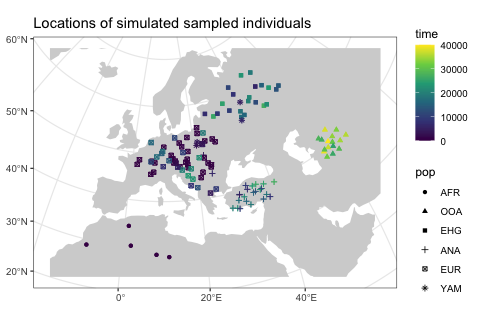Because sf simple features objects (and, by extension, even slendr_spatial objects) are internally stored as normal data frames with couple more bells and whistles on top of them, we have all the powerful tools for manipulating tabular data at our disposal.

As an example, let’s say we wanted to split the sampled individuals in the tree sequence into epochs and plot those individually using standard ggplot2 features. We could simply first do this, adding a new column specifying to which epoch does each simulated individual belong:

epochs <- sampled_data %>%
mutate(epoch = cut(time, breaks = c(40000, 30000, 10000, 4000, 0)),
epoch = ifelse(is.na(epoch), 0, epoch),
epoch = factor(epoch, labels = c("present", "(present, 4 ky]", "(4 ky, 10 ky]",
"(10 ky, 30 y]", "(30 ky, 40 ky]")))

This chunk of code simply adds a new column epoch to the sf spatial data frame object called epochs here.

Then we can use the ggplot2 function geom_sf to plot the locations of sampled individuals on the map, with each facet corresponding to one epoch (the warning can be safely ignored):

ggplot() +
geom_sf(data = map, fill = "lightgray", color = NA) +
geom_sf(data = epochs, aes(shape = pop, color = pop)) +
facet_wrap(~ epoch) +
ggtitle("Locations of simulated sampled individuals in different epochs") +
theme_bw()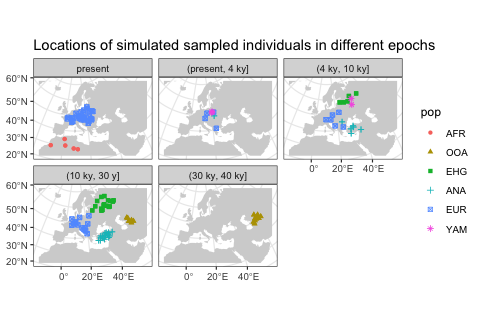We hope this little excursion to handling slendr spatial objects (and, by extension, sf objects) with standard data frame manipulation functions and ggplot2 visualisation convinced you that you have a great flexibility in analysing spatial slendr data. For best introduction into so-called “tidy” data analysis, we encourage you to read the freely-available book R for Data Science.

## Extracting spatio-temporal ancestral relationships

Perhaps even more useful than plotting the locations of simulated individuals is accessing the locations (and times) of all ancestors of a particular tree sequence node (a “focal node”). Starting from the focal node or individual, we can trace the geographical location of nodes in its lineage going back all the way to the root with the function ts_ancestors().

Because we record the time and the location of every individual that happens to be the ancestor of at least one sampled individual, this means that we know the true location of every node of the tree sequence.

The simplest use case is determining the locations and times of every single node in the genealogical history of an individual along the tree sequence (it is possible to recover ancestral relationships for multiple samples at once too):

ind <- "EUR_51"

lineages <- ts_ancestors(ts, ind, verbose = TRUE)
#> Collecting ancestors of EUR_51 [1/1]...
#>
#> Generating data about spatial relationships of nodes...

The function starts at a given node (or, if a name of a sampled diploid individual is provided, two nodes), extracts information about all the parent nodes of that node in the entire tree sequence, records their locations and times, then proceeds one level “higher” in the genealogical history to gather information about the parents of those parent nodes, etc., until it reaches a root node. The result of this process is another sf object in which each row of the table encodes information about single branch in the genealogy of the “focal” node or individual (in our example, "EUR_25"):

lineages
#> Simple feature collection with 200 features and 12 fields
#> Active geometry column: connection
#> Geometry type: LINESTRING
#> Dimension:     XY
#> Bounding box:  xmin: 2119570 ymin: 380221.3 xmax: 8487224 ymax: 4585328
#> Projected CRS: ETRS89-extended / LAEA Europe
#> # A tibble: 200 × 15
#>    name   pop   node_id level child_id parent_id child…¹ paren…² child…³ paren…⁴
#>  * <chr>  <fct>   <int> <fct>    <int>     <int>   <dbl>   <dbl> <fct>   <fct>
#>  1 EUR_51 EUR       242 1          242       265       0    1330 EUR     EUR
#>  2 EUR_51 EUR       242 2          265       283    1330    3070 EUR     EUR
#>  3 EUR_51 EUR       242 2          265       326    1330   10240 EUR     EUR
#>  4 EUR_51 EUR       242 3          283       284    3070    3100 EUR     EUR
#>  5 EUR_51 EUR       242 3          326       352   10240   15010 EUR     EUR
#>  6 EUR_51 EUR       242 4          284       288    3100    3850 EUR     EUR
#>  7 EUR_51 EUR       242 4          352       354   15010   15250 EUR     EUR
#>  8 EUR_51 EUR       242 4          352       354   15010   15250 EUR     EUR
#>  9 EUR_51 EUR       242 5          288       309    3850    6820 EUR     EUR
#> 10 EUR_51 EUR       242 5          354       358   15250   15670 EUR     EUR
#> # … with 190 more rows, 5 more variables: child_location <POINT [m]>,
#> #   parent_location <POINT [m]>, connection <LINESTRING [m]>, left_pos <dbl>,
#> #   right_pos <dbl>, and abbreviated variable names ¹​child_time, ²​parent_time,
#> #   ³​child_pop, ⁴​parent_pop

In each row of the table, two columns location and parent_location carry the spatial location of a node (node_id) and its parent node (parent_id), respectively, same with the columns time and parent_time (times of nodes) and pop and parent_pop (populations to which the nodes belong). The column connection contains an sf geometry object of the line connecting the two nodes in the coordinate reference system of the “model world”. The column focal_id tells us to which focal node’s genealogy the rows of the table belong to, and the level column shows how deep in the genealogical past does each branch (i.e. row of the table) belong to.

This table contains a complete information about spatio-temporal relationships between the nodes in the genealogy of the given focal sample. In the spirit of demonstrating of slendr tree sequence tables interact with the sf and ggplot2 environments, let’s look at the most immediate parent nodes of the two nodes in the sampled individual (i.e. nodes at the level 1) using the filter function from the R package dplyr:

filter(lineages, level == 1)
#> Simple feature collection with 2 features and 12 fields
#> Active geometry column: connection
#> Geometry type: LINESTRING
#> Dimension:     XY
#> Bounding box:  xmin: 4549354 ymin: 2660139 xmax: 4896183 ymax: 3001147
#> Projected CRS: ETRS89-extended / LAEA Europe
#> # A tibble: 2 × 15
#>   name   pop   node_id level child_id parent_id child_…¹ paren…² child…³ paren…⁴
#> * <chr>  <fct>   <int> <fct>    <int>     <int>    <dbl>   <dbl> <fct>   <fct>
#> 1 EUR_51 EUR       242 1          242       265        0    1330 EUR     EUR
#> 2 EUR_51 EUR       243 1          243       259        0     730 EUR     EUR
#> # … with 5 more variables: child_location <POINT [m]>,
#> #   parent_location <POINT [m]>, connection <LINESTRING [m]>, left_pos <dbl>,
#> #   right_pos <dbl>, and abbreviated variable names ¹​child_time, ²​parent_time,
#> #   ³​child_pop, ⁴​parent_pop

As we mentioned above, there are three columns encoding spatial information: location and parent_location carry information about the location of the child and parent node (POINT class), and the connection object (LINESTRING class) contains the line connecting the two nodes (both a branch in the tree sequence and also the spatial connection). We can plot all three spatial features (two points and a line) individually on a map:

level1_branches <- ts_ancestors(ts, "EUR_10") %>% filter(level == 1)

ggplot() +
geom_sf(data = map, fill = "lightgray", color = NA) +
geom_sf(data = level1_branches[, ]$child_location, shape = 13, size = 3, color = "red") + geom_sf(data = level1_branches[, ]$parent_location, shape = 20, color = level1_branches[, ]$node_id) + geom_sf(data = level1_branches[, ]$connection, linetype = 3) +
theme_bw() +
ggtitle("Parent nodes (blue) of a focal individual (red)")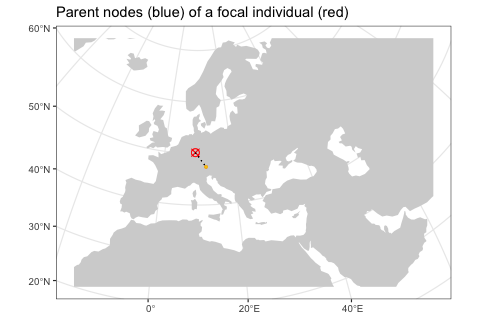In the figure above we can see the red focal node and its immediate parents in the tree sequence genealogy (in the coalescent sense, not immediate parents of that individual!).

A more convenient way to do this analysis is a companion function to ts_ancestors() called plot_ancestors(). This function accepts an sf object with the spatial branching data created by ts_ancestors() and plots the paths between nodes on a map leading from a focal node up to the root(s) of the tree sequence (instead of just paths to immediate parents shown in the previous figure). In this case, because we are working with a single diploid individual, we get two sets of paths for each of its nodes (chromosomes) and plot them in two facets:

ggplot() +
geom_sf(data = map) +
geom_sf(data = lineages, size = 0.5, aes(alpha = parent_time)) +
geom_sf(data = sf::st_set_geometry(lineages, "parent_location"),
aes(shape = parent_pop, color = parent_pop)) +
geom_sf(data = filter(ts_nodes(ts), name == ind), size = 3) +
guides(alpha = "none") +
coord_sf(expand = 0) +
labs(x = "longitude", y = "latitude") +
facet_grid(. ~ node_id)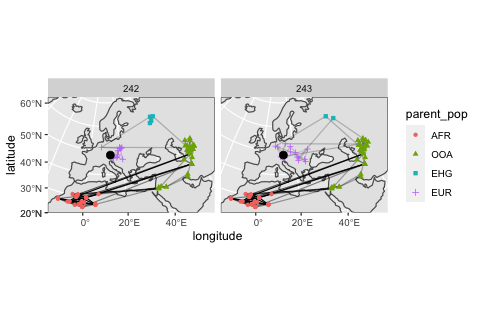You can compare this result to the animation which recapitulates the simulation, presented in the first vignette.

After comparing our spatial tree sequence figure with the animation, we can immediately notice several things:

1. All spatial tree sequence paths trace the ancestry of our single European individual back to Africa. In fact, we also see a cluster of past ancestral nodes (i.e. concentrated coalescent events) in the place where the Out of Africa (OOA) migrant population settled around 40,000 thousand years ago (yellow population in the the animation).

2. The first chromosome traces its ancestry to the Yamnaya population indicated by a star. This is expected because we programmed Yamnaya to contribute significant part of ancestry to present-day Europeans (compare to the demographic graph on top of this vignette). In turn, the Yamnaya node traces its ancestry to EHG (which is its parental population). We can see a cluster of EHG nodes in one location because we did not specify any spatial dynamic for this population in our model

3. We also see that the other chromosome traces of its ancestry to the Anatolia (triangles). This makes sense, because we have simulated European ancestry as being part Anatolian.

Let’s look at the spatial ancestry of another sample. For instance, we know that the simulated history of the Anatolian population in our model is much simpler. According to the demographic graph above, Anatolians split from the ancestral population of Eurasians in Anatolia and then expanded in a wave to Europe. We have sampled the following individuals:

ts_samples(ts) %>% filter(pop == "ANA")
#> # A tibble: 24 × 3
#>    name    time pop
#>    <chr>  <int> <chr>
#>  1 ANA_1  27000 ANA
#>  2 ANA_2  26000 ANA
#>  3 ANA_3  25000 ANA
#>  4 ANA_4  24000 ANA
#>  5 ANA_5  23000 ANA
#>  6 ANA_6  22000 ANA
#>  7 ANA_7  21000 ANA
#>  8 ANA_8  20000 ANA
#>  9 ANA_9  19000 ANA
#> 10 ANA_10 18000 ANA
#> # … with 14 more rows

Can we see a hint of the spatial dynamics of Anatolians in the spatio-temporal distribution of ancestral node locations of one of the sampled individuals? Let’s pick the last individual and immediately plot its spatial ancestry tidyverse-style using the pipe operator %>%:

lineages <- ts_ancestors(ts, "ANA_21")

ggplot() +
geom_sf(data = map) +
geom_sf(data = lineages, size = 0.5, aes(alpha = parent_time)) +
geom_sf(data = sf::st_set_geometry(lineages, "parent_location"),
aes(shape = parent_pop, color = parent_pop)) +
geom_sf(data = filter(ts_nodes(ts), name == "ANA_21"), size = 3) +
guides(alpha = "none") +
coord_sf(expand = 0) +
labs(x = "longitude", y = "latitude") +
facet_grid(. ~ node_id)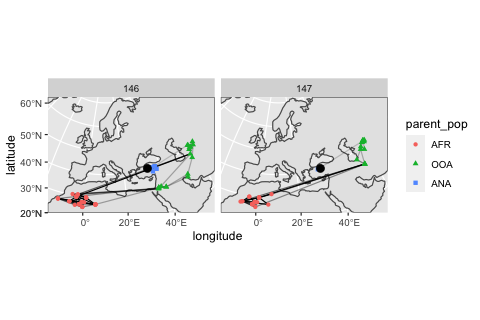As we might expect given the late age of the sample, its position in the map above (red crossed circle) is not in Anatolia but in Europe because it represents one of the descendants of migrants who moved from Anatolia into Europe. This can be clearly seen in the position of its parental nodes in the tree sequence: these nodes represent real individuals who lived at some point in the past, and we can see that they did, indeed, lived in Anatolia.

## Calculating distances and other statistics using the sf package

How can we summarise the spatial ancestral dynamics in the figures using some statistics?

Lets take one more look at the sf object with the locations and times of ancestral nodes of sampled individuals, focusing on the following subset of columns:

lineages <-
ts_samples(ts) %>%
pull(name) %>%
ts_ancestors(ts, x = .)

select(lineages, connection, child_time, parent_time)
#> Simple feature collection with 16462 features and 2 fields
#> Geometry type: LINESTRING
#> Dimension:     XY
#> Bounding box:  xmin: 2119570 ymin: 380221.3 xmax: 8576763 ymax: 4902147
#> Projected CRS: ETRS89-extended / LAEA Europe
#> # A tibble: 16,462 × 3
#>                             connection child_time parent_time
#>                       <LINESTRING [m]>      <dbl>       <dbl>
#>  1  (8158881 3288546, 8150563 1777187)      40000       44560
#>  2  (8158881 3288546, 7116257 1612158)      40000       47530
#>  3  (8150563 1777187, 7140201 1312578)      44560       48340
#>  4  (7116257 1612158, 7140201 1312578)      47530       48340
#>  5  (7116257 1612158, 7140201 1312578)      47530       48340
#>  6  (7140201 1312578, 6905379 1333523)      48340       49180
#>  7  (7140201 1312578, 6795742 1274583)      48340       49600
#>  8  (7140201 1312578, 6795742 1274583)      48340       49600
#>  9 (7140201 1312578, 2119570 772520.6)      48340       71350
#> 10  (6905379 1333523, 6795742 1274583)      49180       49600
#> # … with 16,452 more rows

We can use standard dplyr table manipulation functions to compute the distances between connected notes and the times that separate them (i.e. branch lengths in the traditional phylogenetic sense). We can then use those two quantities to compute how fast (if any at all) was the movement between ancestral individuals in different time periods of the history of a sample:

distances <- lineages %>%
mutate(branch_length = abs(parent_time - child_time) / model$generation_time, distance = sf::st_length(connection) %>% units::set_units(km) %>% as.numeric(), speed = distance / branch_length, epoch = cut(parent_time, breaks = c(Inf, seq(60000, 0, by = -3000)), dig.lab = 10, include.lowest = TRUE)) %>% as_tibble() %>% # strip away the spatial annotation select(name, pop, node_id, branch_length, distance, speed, parent_pop, parent_time, child_pop, child_time, epoch) Let’s also convert the data (absolute distances or distance per generation – i.e., the “speed”) into a long format for easier plotting side by side: distances_long <- distances %>% filter(child_time < 60000) %>% filter(!pop %in% c("AFR", "OOA")) %>% tidyr::pivot_longer(cols = c(distance, speed), names_to = "stat", values_to = "value") %>% mutate(facet = case_when( stat == "distance" ~ "absolute distance of a node from parent", stat == "speed" ~ "distance traveled by a node per generation")) Let’s try to summarise the information about distances “traveled” by nodes in different time period by fitting a spline (rather than plotting the raw data with individual nodes): distances_long %>% ggplot(aes(child_time, value, color = child_pop)) + geom_smooth(method = "loess", aes(group = child_pop)) + geom_hline(yintercept = 0, linetype = 2, size = 0.5) + labs(y = "kilometers", x = "time [years ago]") + theme(axis.text.x = element_text(hjust = 1, angle = 45), legend.position = "bottom") + facet_wrap(~ facet, scales = "free_y") + guides(color = guide_legend("ancestral node population")) #> geom_smooth() using formula 'y ~ x' #> Warning in simpleLoess(y, x, w, span, degree = degree, parametric = #> parametric, : pseudoinverse used at 54022 #> Warning in simpleLoess(y, x, w, span, degree = degree, parametric = #> parametric, : neighborhood radius 3527.6 #> Warning in simpleLoess(y, x, w, span, degree = degree, parametric = #> parametric, : reciprocal condition number 1.9309e-29 #> Warning in simpleLoess(y, x, w, span, degree = degree, parametric = #> parametric, : There are other near singularities as well. 1.2444e+07 #> Warning in predLoess(object$y, object$x, newx = if #> (is.null(newdata)) object$x else if (is.data.frame(newdata))
#> as.matrix(model.frame(delete.response(terms(object)), : pseudoinverse used at
#> 54022
#> Warning in predLoess(object$y, object$x, newx = if
#> (is.null(newdata)) object$x else if (is.data.frame(newdata)) #> as.matrix(model.frame(delete.response(terms(object)), : neighborhood radius #> 3527.6 #> Warning in predLoess(object$y, object$x, newx = if #> (is.null(newdata)) object$x else if (is.data.frame(newdata))
#> as.matrix(model.frame(delete.response(terms(object)), : reciprocal condition
#> number 1.9309e-29
#> Warning in predLoess(object$y, object$x, newx = if
#> (is.null(newdata)) object$x else if (is.data.frame(newdata)) #> as.matrix(model.frame(delete.response(terms(object)), : There are other near #> singularities as well. 1.2444e+07 #> Warning in simpleLoess(y, x, w, span, degree = degree, parametric = #> parametric, : pseudoinverse used at 54022 #> Warning in simpleLoess(y, x, w, span, degree = degree, parametric = #> parametric, : neighborhood radius 3527.6 #> Warning in simpleLoess(y, x, w, span, degree = degree, parametric = #> parametric, : reciprocal condition number 1.9309e-29 #> Warning in simpleLoess(y, x, w, span, degree = degree, parametric = #> parametric, : There are other near singularities as well. 1.2444e+07 #> Warning in predLoess(object$y, object$x, newx = if #> (is.null(newdata)) object$x else if (is.data.frame(newdata))
#> as.matrix(model.frame(delete.response(terms(object)), : pseudoinverse used at
#> 54022
#> Warning in predLoess(object$y, object$x, newx = if
#> (is.null(newdata)) object$x else if (is.data.frame(newdata)) #> as.matrix(model.frame(delete.response(terms(object)), : neighborhood radius #> 3527.6 #> Warning in predLoess(object$y, object$x, newx = if #> (is.null(newdata)) object$x else if (is.data.frame(newdata))
#> as.matrix(model.frame(delete.response(terms(object)), : reciprocal condition
#> number 1.9309e-29
#> Warning in predLoess(object$y, object$x, newx = if
#> (is.null(newdata)) object\$x else if (is.data.frame(newdata))
#> as.matrix(model.frame(delete.response(terms(object)), : There are other near
#> singularities as well. 1.2444e+07